Free Printable Worksheets for Fourth grade

## Order of Operations I (Set 3)Download this worksheet for students learning to simplify expressions using the correct order of operations. The problems use addition, subtraction, multiplication and division....

## Order of Operations I (Set 2)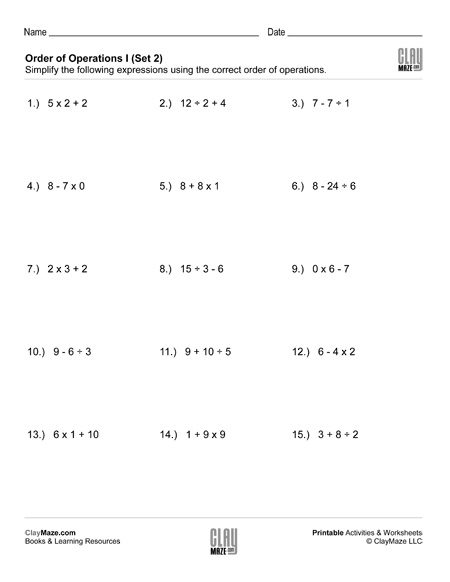This worksheet is great for students learning to use the correct order of operations to simplify expressions.  The problems involve 3 numbers using addition or subtraction combined with...

## Order of Operations I (Set 1)This is a basic worksheet for practice with simplifying expressions using the correct order of operations. Each problem uses 3 numbers involving addition or subtraction combined with...

## Reading Comprehension Word Problem: Pizza Party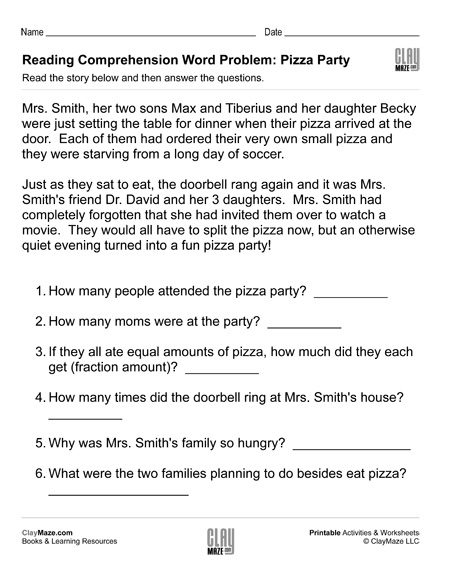As our subject matter becomes more and more technical and complex, it becomes increasingly important for children to learn to read carefully and re-read a passage in order to fully grasp the...

## Crossword Puzzle Research Project – Branches of Science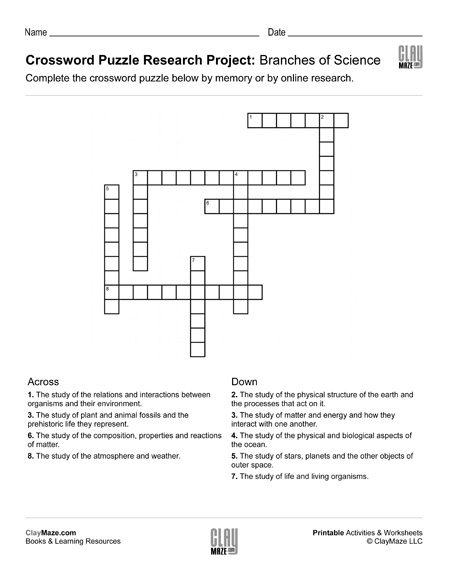This is a little bit of a spin from a traditional Crossword Puzzle lesson.   This worksheet can be used as a followup to some study on the branches of science or it can be used as an online resea...

## Crossword Puzzle Research Project – Animal Classifications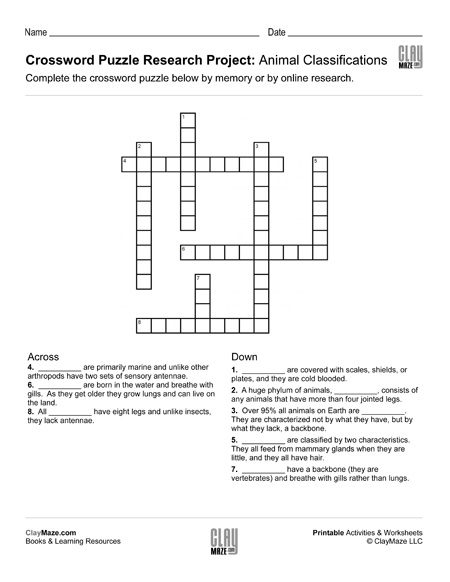This crossword puzzle worksheet can be used as a stand-alone lesson or quiz for students more accustomed with Animal Classifications or used as an online research project for those with less exposu...

## Long Division Worksheet with Double Digit Divisors (Set 4)Download this long division practice worksheet. It features double digit divisors and no remainders. – 9 problems...

## Long Division Worksheet with Double Digit Divisors (Set 3)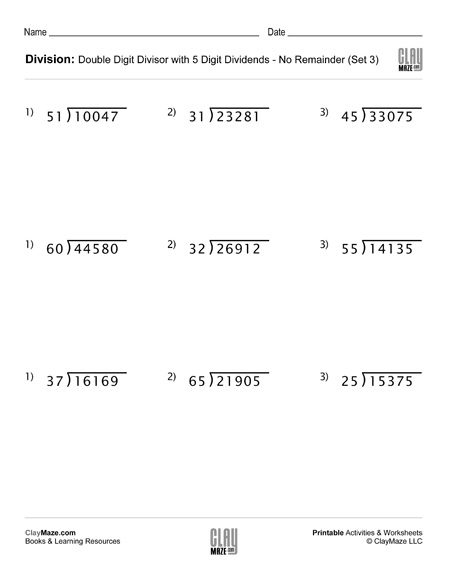Use this worksheet for pracice with long division. The divisors have 2 digits and there are no remainders. – 9 problems...

## Long Division Worksheet with Double Digit Divisors (Set 2)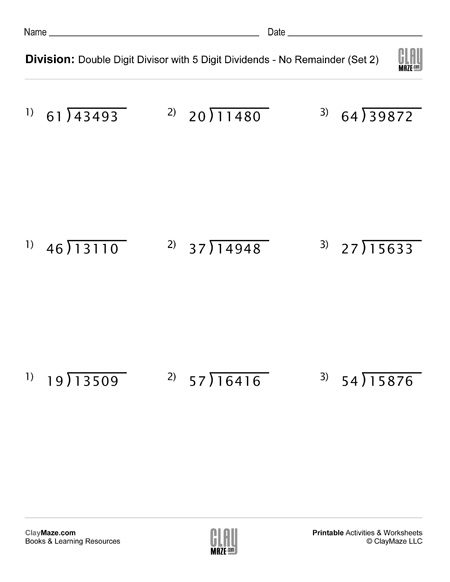This division worksheet is on long division with double digit divisors. There are no remainders. – 9 problems...

## Long Division Worksheet with Double Digit Divisors (Set 1)This worksheet is a set of long division problems with double digit divisors. There are no remainders. – 9 problems...

## Division Worksheet with Single Digit Divisors (Set 4)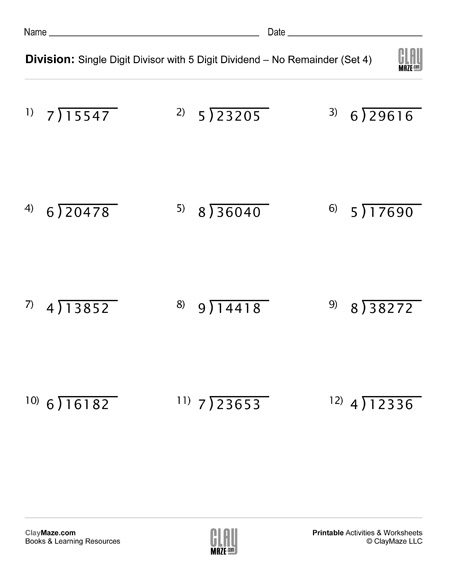Use this worksheet for division practice with single digit divisors. The problems can be solved using either long division or short division. There are no remainders....

## Division Worksheet with Single Digit Divisors (Set 3)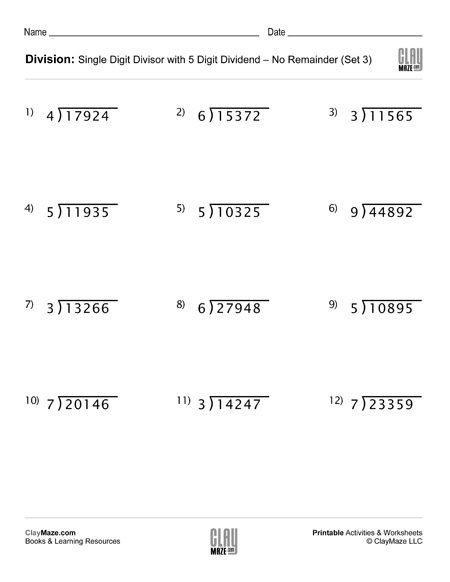This is a worksheet on division practice with a single digit divisor. They can be done using short division or long division. There is plenty of room for either. – No remainders....

## Division Worksheet with Single Digit Divisors (Set 2)This division worksheet can be completed by using either long division or short division. Each problem has a 5-digit dividend and a single digit divisor. – No remainders....

## Division Worksheet with Single Digit Divisors (Set 1)This is a division worksheet with 5 digit dividends and single digit divisors. They can be solved by either short division or long division. There are no remainders....

## General Statistics Worksheet – DThis worksheet gives a data set that should be used to complete the graph and answer the questions. The student can refer to the statistics terms defined at the top of the worksheet to answer the...